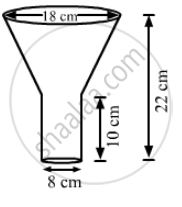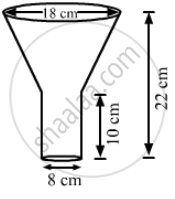Advertisement Remove all ads

# An Oil Funnel Made of Tin Sheet Consists of a 10 Cm Long Cylindrical Portion Attached to a Frustum of a Cone. If the Total Height is 22 Cm, Diameter of the - Mathematics

Sum

An oil funnel made of tin sheet consists of a 10 cm long cylindrical portion attached to a frustum of a cone. If the total height is 22 cm, diameter of the cylindrical portion is 8 cm and the diameter of the top of the funnel is 18 cm, then find the area of the tin sheet required to make the funnel.Advertisement Remove all ads

#### SolutionWe have,

Height of the cylindrical portion, h = 10 cm,

height of the frustum of cone portion, H = 22 - 10 = 12 cm,

Radius of the  cylindrical portion = Radius of smaller end of frustum portion,

Radius of larger end of frustum portion, R = 18/2 = 9 cm

Also, the slant height of the frustum, l = sqrt(("R - r")^2 + "H"^2)

=sqrt((9 - 4)^2+12^2

=sqrt(5^2+12^2)

=sqrt(25+144

=sqrt(169)

=13  "cm"

Now,

The area of the tin sheet required = CSA of frustum of cone + CSAA of cylinder

= π (R + r) l + 2πrh

= 22/7xx(9+4)x13+2xx22/7xx4xx10

=22/7xx13xx13+22/7xx80

= 22/7xx(169+80)

=22/7xx249

≈ 782.57 cm

So, the area of the tin sheet required to make the funnel is 782.57 cm2.

Is there an error in this question or solution?
Advertisement Remove all ads

#### APPEARS IN

RS Aggarwal Secondary School Class 10 Maths
Chapter 19 Volume and Surface Area of Solids
Exercise 19C | Q 21 | Page 912
Advertisement Remove all ads

#### Video TutorialsVIEW ALL 

Advertisement Remove all ads
Share
Notifications

View all notifications

Forgot password?
Course